Home

# ON POLYTOPAL UPPER BOUND SPHERES

## Abstract

Generalizing a result (the case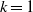$k= 1$ ) due to M. A. Perles, we show that any polytopal upper bound sphere of odd dimension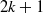$2k+ 1$ belongs to the generalized Walkup class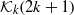${ \mathcal{K} }_{k} (2k+ 1)$ , i.e., all its vertex links are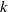$k$ -stacked spheres. This is surprising since it is far from obvious that the vertex links of polytopal upper bound spheres should have any special combinatorial structure. It has been conjectured that for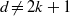$d\not = 2k+ 1$ , all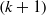$(k+ 1)$ -neighborly members of the class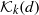${ \mathcal{K} }_{k} (d)$ are tight. The result of this paper shows that the hypothesis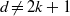$d\not = 2k+ 1$ is essential for every value of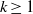$k\geq 1$ .

## References

Hide All
MathJax
MathJax is a JavaScript display engine for mathematics. For more information see http://www.mathjax.org.

# ON POLYTOPAL UPPER BOUND SPHERES

## Metrics

### Full text viewsFull text views reflects the number of PDF downloads, PDFs sent to Google Drive, Dropbox and Kindle and HTML full text views.

Total number of HTML views: 0
Total number of PDF views: 0 *Loading metrics...

### Abstract viewsAbstract views reflect the number of visits to the article landing page.

Total abstract views: 0 *Loading metrics...

* Views captured on Cambridge Core between <date>. This data will be updated every 24 hours.

Usage data cannot currently be displayed